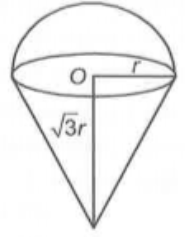# A uniform solid hemisphere of radius r is joined to a uniform solid right circular cone of base radius r and height √3r. If both have same density, then find the position of centre of mass from centre of hemisphereKhareedo DN Pro and dekho sari videos bina kisi ad ki rukaavat ke!

उत्तर

Step by step solution by experts to help you in doubt clearance & scoring excellent marks in exams.

Transcript

radius R is going right circular cone of base radius R and height under root 3 are both have same density and then find the position of centre of from the centre so you can see that this is a solid hemisphere and this is a solid right circular cone of radius R is the radius of base of the videos of hemisphere are made up of a material that is they are having same density have to find where is the centre of mass located in very easy we can see the main concern that we are going to use it and let us take this is as x-axis and let us take this as y axis
latest take this as origin at from origin we will find the centre of masses of this combined solid now we know that for this hemisphere its centre of mass if it has uniform mass density write on Mars is uniformly distributed then it has its centre of mass and this distance is simply 38 what is the coordinates of this point this will be zero Hour 3 hour by 8 where R is the radius of this hemisphere now similarly the centre of mass for this cone in will be in this negative direction and it is at a height from this point it is at a height H by 4 the coordinates here will be simply 0 - why because this is low the why this is below the origin right so this is positive y-axis and this is negative y axis
this is simply H by 4 this is simply as my 4C to is a touch my phone the simply root 3 hours so this will be root 3 divided by 4 now this is the position of centre of mass from this figure 1 thing is very very clear that the X co-ordinate of centre of mass will be simply 0y because the centre of masses lie whenever the centre of mass of line in a straight line then the common centre of mass will be somewhere along this line exponent will be simply zero now we have to find what is the y-coordinate of centre what is the formula for finding Y coordinates of centre of letters take the mass of this as M1 and let us take the mass of this as M2 so very simple and 1 by 1 + M2 why to hold
/ M1 vs M2 but it is given in the question that their densities are same same density rho and their densities are equal then we can find out the masses mass of this hemisphere will be simply equals to density into its volume what is the volume of a 35 Rs the radius of the semester answer to why 35 are you this is the mass now let us take the mass of the cone again the density is it is simply what is the volume of the cone volume of the cone is simply one upon 35ah square h r square and the height hair simply square root 3 and small letters are going to get
now we find by sea refined why are substituted C 1 is a p h b o b f x 38 this is the value of Y and this is the value of this and what is the density will cancel out from everywhere the density so that you can see that the PI is cancel out from all the time now you can see that is common rights are you will also cancel out from all the terms this are you will
simply cancel out now latest cancel out the other terms one upon the latest write the remaining letter write the remaining the device will be simply by 4 minus 3 by 4 / Tu plus square root 3 now you can see the numerator numerator simply cancel out so from here what you get the value of centre of mass of solid is what is the meaning is at the centre of mass and centre of react to find the position of centre of mass of the centre of any space it lies at the centre of the correct

संबंधित वीडियो